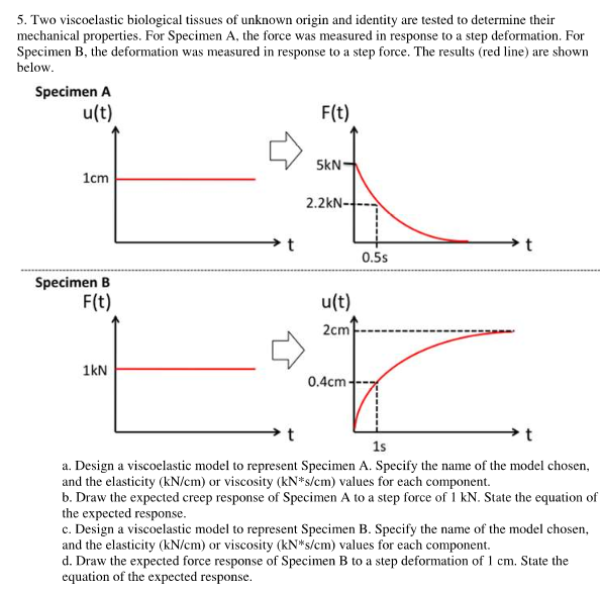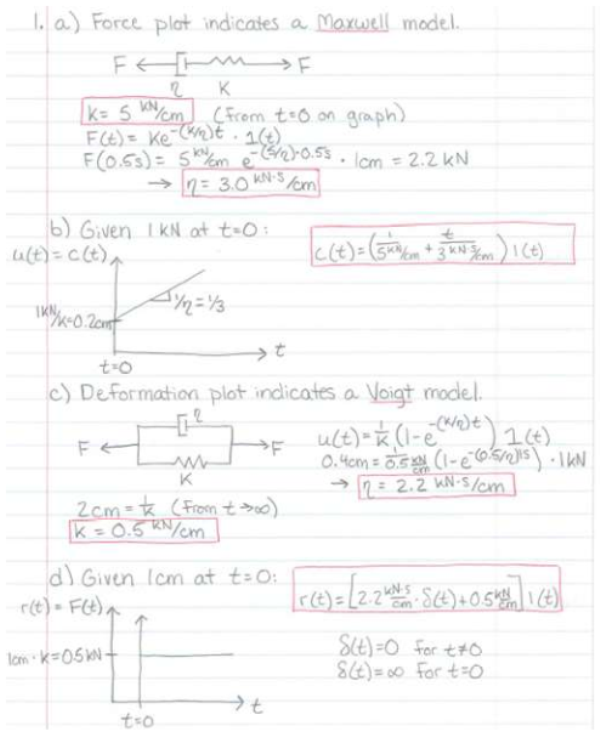Two viscoelastic biological tissues of unknown origin and identity are tested to determine their mechanical properties. For specimen A, the force was measured in reponse to a step deformation. for specimen B, the deformation was measured in response to a step force. The results (red line) are shown below. a) Design a viscoelastic model to represnt Specimen A. Specify the name of the model chosen, and the elasticity or viscosity values for each component. b) Draw the expected creep response of specimen A to a step force of 1 kN. State the equation of the expected response. c) Design a viscoelastic model to represent specimen B. Specify the name of the model chosen, and the elasticity or viscosity values for each component. d) Draw the expected force reponse of specimen B to a step deformation of 1 cm. State the equation of the expected response.Two viscoelastic biological tissues of unknown origin and identity are tested to determine their mechanical properties. For specimen A, the force was measured in reponse to a step deformation. for specimen B, the deformation was measured in response to a step force. The results (red line) are shown below. a) Design a viscoelastic model to represnt Specimen A. Specify the name of the model chosen, and the elasticity or viscosity values for each component. b) Draw the expected creep response of specimen A to a step force of 1 kN. State the equation of the expected response. c) Design a viscoelastic model to represent specimen B. Specify the name of the model chosen, and the elasticity or viscosity values for each component. d) Draw the expected force reponse of specimen B to a step deformation of 1 cm. State the equation of the expected response.

biomechanics page 1 biomechanics biomechanics biomechanics biomechanics biomechanics biomechanics biomechanics biomechanics page 2